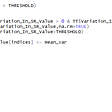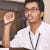# Analysis of Time Series Data— Lecture 02

In this lecture, we will study analysis of Time series data.

Step -01: Read data and make sure that you convert date column datatype to DateTime object.

`data = pd.read_csv('airline-passenger-traffic.csv', header = None)data.columns = ['Month','Passengers']data['Month'] = pd.to_datetime(data['Month'], format='%Y-%m')data = data.set_index('Month')data.head(12)`

Step-02: Plot Time Series data

`data.plot(figsize=(12, 4))plt.legend(loc='best')plt.title('Airline passenger traffic')plt.show(block=False)`

Observation from above graph:

• Traffic increasing year on year
• Pattern repeat after every year, follow summer → winter trend
• Some data is missing in year — 1951,1954 and 1960 and reason can be data capture issue or data was not recorded as the event did not occur. For example, if sales did not happen on x date may be due to some operational issues, the record entry of sales would be 0 on that particular date.

Step — 03: Handling Missing values

• Mean Imputation: Imputing the missing values with the overall mean of the data.
`data = data.assign(Passengers_Mean_Imputation=data.Passengers.fillna(data.Passengers.mean()))data[['Passengers_Mean_Imputation']].plot(figsize=(12, 4))plt.legend(loc='best')plt.title('Airline passenger traffic: Mean imputation')plt.show(block=False)`

Imputing the missing value with mean, median and mode can reduce the variance. Not suggested in Time Series data.

• Last observation carried forward: We impute the missing values with its previous value in the data.
`data['Last_observation_carried_forward'] = data['Passengers'].ffill()`

Imputing the missing value with the next observed value and last observed value can introduce bias in analysis and perform poorly when data has a visible trend.

• Linear interpolation: You draw a straight line joining the next and previous points of the missing values in the data.
`data['Passengers_Linear_Interpolation'] = data.assign(Passengers_Linear_Interpolation=data.Passengers.interpolate(method='linear'))`

To deal with missing values in time series data with trends is Linear interpolation as it imputes the missing value with the average of previous and next values.

Step — 04: Decomposition of Time Series

WIP !!

--

--

--

## More from everything about forecasting

Everything about Forecasting (Zero to Hero)

## The Basics of Predictive Analytics## Logic Behind The Drop## Reducing the carbon footprint by controlling traffic lights using Python## Soiling Analysis — Solar PV Modules## An analysis of daily mortality in France during the COVID19 pandemic## Missed opportunities in the EU’s revised open data and re-use of public sector information…## Aakash Goel

Senior Data Scientist @ Fractal Analytics

## Getting started with Sentiment Analysis using Pre-trained NLP Models with python codes## Predicting GDP of Georgia using LSTM## Handling Imbalanced Datasets by Oversampling and Undersampling with Python Implementation## Implementing Decision Trees: Mathematically and Using Python